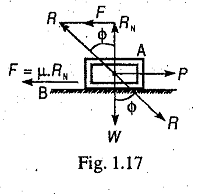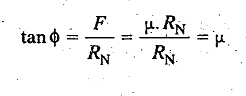# Limiting Angle of Friction

Limiting Angle of Friction is defined as the angle which the resultant reaction (R) makes with the normal reaction (RN).Consider a body A of weight W resting on a horizontal plane B as shown in Fig. 1.17. If a horizontal force P is applied to the body, no relative motion takes place until the applied force P is equal to the force of friction F, acting opposite to the direction of motion. The magnitude of this force of friction is
F = µ W = µ.RN, where RN is the normal reaction.

In the limiting case, when the body just begins to move, it is in equilibrium under the action of the following three forces :

1. Weight of the body (W),
2. Applied horizontal force (P), and
3. Reaction (R) between the body A and the plane B.

The reaction R must, therefore,be equal and opposite to the resultant of W and P and will be inclined at an angle (ϕ) to the normal reaction (RN). This angle ϕ is called the limiting angle of friction or simply angle of friction. From Fig. 1.17, we find that### You may like these posts:

1.What is the physical significance of limiting of friction?

1.similar to the co efficient of friction , the tan of angle of limiting friction shows another co efficient

2.What is the physical significance of limiting of friction?

1.Hey ben johns. Im a novis at this stuff but limiting friction is significant as it is the max. possible friction btw 2 surfaces so im guessing calculations can be made using this max friction for safety certainty.

2.Idk much but i think Limiting fricion is the max possible friction so this value is impt for calculation for safety certainty

3.What is R...it is resultant btw Rñ&F or btw W&P??

4.I think this will help to decide the curvature during road construction and like versa